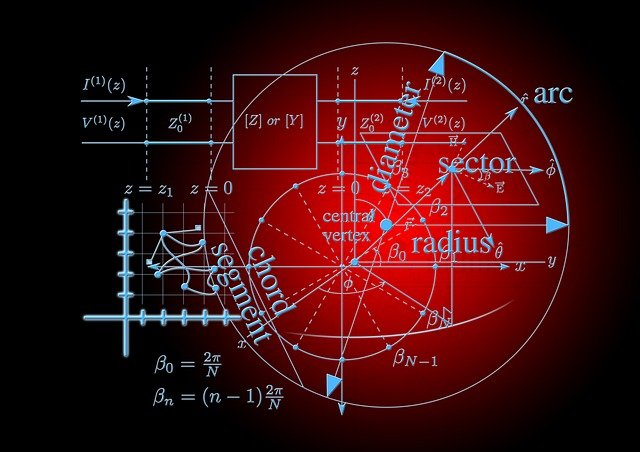Digital Tbucket Tank (DTT)

# Pioneering research by Polish mathematicians on the symmetry of all symmetriesPolish mathematicians have managed to solve an important problem regarding the Symmetry of all symmetries to solve. This was an unsolved problem for several decades - one of the greatest challenges of the geometric theory of groups.

The results of Dr. Marek Kaluba (Adam Mickiewicz University and Karlsruhe Institute of Technology), Prof. Dawid Kielak (University of Oxford) and Prof. Piotr Nowak (Mathematical Institute of the Polish Academy of Sciences) were published in one of the most renowned mathematical journals Annals of Mathematics published.

Image source: Pixabay

We have solved a particular long open problem by showing that a particular infinite family of algebraic objects - groups - has the property T and is therefore very incompatible with the Euclidean geometry is ", sums up Nowak.

And dr. Marek Kaluba adds: Thanks to our research, we have understood certain geometrical aspects of groups that all encode symmetries.
The objects with the Property Tthat we examined have very exotic geometric properties (they cannot be called Symmetries in der Euclidean geometry will be realized). Does this seem disconnected from reality? On the surface, yes. But knowledge of this complicated property of T has already found application. It enables, for example, the construction of expanders - graphs with a large number of connections, which can be found in Streaming algorithms be used. And such algorithms are among other things for the display of Trends on Twitter responsible.

The question of whether the groups we studied have such a property T appeared in print in the 90s. When I was a PhD student, that was a problem that I come across at every other lecture and conference Group theory heard - summarizes Piotr Nowak.
And Dawid Kielak adds: Our result explains how a certain algorithm works. It is the product replacement algorithm that is used when you want to pull items from a large set, e.g. B. a set with more elements than the number of particles in the universe. This one algorithm It's been around since the 1990s and it works a lot better than expected. Our article explains why it works so well - says Prof. Kielak.

And he adds: Computer science is a new one Physics. What surrounds us are not just particles, but increasingly also algorithms. Our job as mathematicians will be to understand algorithms, to show why they work or not; why they're fast or slow. Scientists have relied on computer calculations for their mathematical proof. The use of computers to prove theorems in mathematics has not previously been considered particularly elegant. The community of theoretical mathematician mostly wrinkled his nose at computers. But here this modern approach worked extremely well.

The computer just did the chore. But it hasn't replaced logic. Our idea was to apply the reduction of an infinite problem to a finite problem - says Prof. Kielak. And Dr. Marek Kaluba adds: We have our problem on one Optimization problem reduced and then for this Optimization Standard tools used - algorithms that engineers use to design components.

So the computer was given the task of finding a matrix that met certain criteria. The machine created a solution, checked how well it met the given conditions, and gradually improved this matrix to achieve the lowest possible error rate. The only question was how small is the margin of error it can achieve; it turned out that the computer's error on the last approximation was very, very small. So the computation of the computer made it possible - with the right ones mathematical arguments - Obtain rigorous evidence.

The one created by the computer Matrix had 4,5 thousand columns and 4,5 thousand rows. Marek Kaluba explains that the problem they were working on was initially too big to be solved by themselves with a supercomputer. So we used the internal symmetries of this problem to make it easier to find a solution - he says. And he explains that an analogous approach can also be used to solve other problems in the field of optimization of objects by geometric Symmetries Marked are. These symmetries (in algebraic form) will also be observable in the optimization problem and can be used for Complexity reduction can be used - says Dr. Kaluba. And he adds: Although we deal with abstract mathematics, we want our software to be useful in technical applications as well.